Courses

# Inverse Laplace & Z-Transform Notes | EduRev

## Electrical Engineering (EE): Inverse Laplace & Z-Transform Notes | EduRev

The document Inverse Laplace & Z-Transform Notes | EduRev is a part of the Electrical Engineering (EE) Course Signals and Systems.
All you need of Electrical Engineering (EE) at this link: Electrical Engineering (EE)

Inverse Laplace Transform:
We know that there is a one to one correspondence between the time domain signal x(t) and its Laplace Transform X(s). Obtaining the signal 'x(t)' when 'X(s)' is known is called Inverse Laplace Transform (ILT). For ready reference , LT and ILT pair is given below :

X(s) = LT { x(t) } Forward Transform

x(t) = ILT { X(s) } The Inverse TransformS

ome of the methods available for obtaining 'x(t)' from 'X(s)' are :

• The complex inversion formulae.
• Partial Fractions.
• Series method.
• Method of differential equations

In general:

If the Laplace Transform of 'x(t)' is 'X(s)' then the Inverse Laplace Transform of 'X(s)' is given by: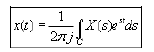Now 'C' is any vertical line in the s-plane that is parallel to the imaginary axis.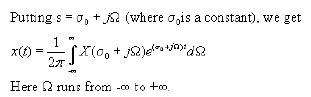Relationship between Laplace Transform and Fourier Transform

The Fourier Transform for Continuous Time signals is infact a special case of Laplace Transform. This fact and subsequent relation between LT and FT are explained below.

Now we know that Laplace Transform of a signal 'x'(t)' is given by: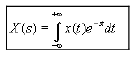The s-complex variable is given by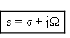But we consider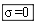and therefore 's' becomes completely imaginary. Thus we have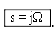. This means that we are only considering the vertical strip at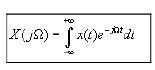From the above discussion it is clear that the LT reduces to FT when the complex variable only consists of the imaginary part . Thus LT reduces to FT along the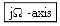(Imaginary axis).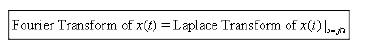Review:

We saw that if the imaginary axis lies in the Region of Convergence of 'X(s)' and the Laplace Transform is evaluated along it.
The result is the Fourier Transform of 'x(t)'.

Relationship between inverse Laplace Transform and inverse Fourier Transform

Similarly while evaluating the Inverse Laplace Transform of 'X(s)' if we take the line ' C ' to be the imaginary axis (provided it lies in the Region of Convergence ). This is shown below as: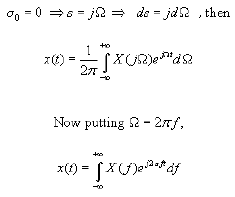Thus above we notice that we get the Inverse Fourier Transform of 'X(f)' as expected.

This tells us that there is a close relationship between the Laplace Transform and the Fourier Transform. In fact the Laplace Transform is a generalization of the Fourier Transform, that is, the Fourier Transform is a special case of the Laplace Transform only. The Laplace Transform not only provides us with additional tools and insights for signals and systems which can be analyzed using the Fourier Transform but they also can be applied in many important contexts in which Fourier Transform is not applicable. For example , the Laplace Transform can be applied in the case of unstable signals like exponential signals growing with time but the Fourier Transform cannot be applied to such signals which do not have finite energy.

Inverse Z - Transform

We know that there is a one to one correspondence between a sequence x[n] and its ZT which is X[z].
Obtaining the sequence 'x[n]' when 'X[z]' is known is called Inverse Z - Transform.
For a ready reference , the ZT and IZT pair is given below.

X[z] = Z { x[n] } Forward Z - Transform

x[n] = Z-1 { X[z] } Inverse Z - Transform

For a discrete variable signal x[n], if its z - Transform is X(z), then the inverse z - Transform of X(z) is given by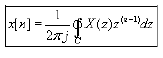where ' C ' is any closed contour which encircles the origin and lies ENTIRELY in the Region of Convergence.

Relationship between Z - Transform and Discrete Time Fourier Transform (DTFT)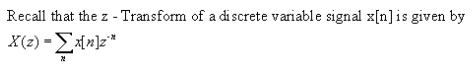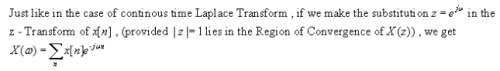Which is same as the Discrete Time Fourier Transform (DTFT) of x[n]. Thus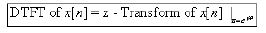Similarly , on making the same substitution in the inverse z - Transform of X(z); provided the substitution is valid , that is, |z|=1lies in the ROC.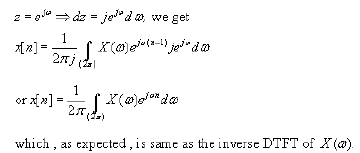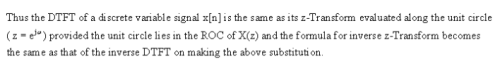Hence we conclude that the z -Transform is just an extension of the Discrete Time Fourier Transform. It can be applied to a broader class of signals than the DTFT, that is, there are many discrete variable signals for which the DTFT does not converge but the z-Transform does so we can study their properties using the z -Transform.

Examples: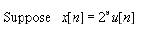The z - Transform of this sequence is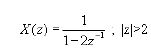Also we observe that the DTFT of the sequence does not exist since the summation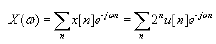'

diverges. This example confirms that in some cases the z - Transform may exist but the DTFT may not.

Conclusion:

In this lecture you have learnt:

• If the Laplace Transform of 'x(t)' is 'X(s)' , then the Inverse Laplace Transform of X(s) is given by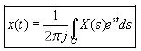where 'C' is any vertical line in the s plane, that is, parallel to the imaginary axis.

• Fourier Transform of 'x(t)' = Laplace Transform of 'x(t)' when s=jw i.e. if the imaginary axis lies in the Region of Convergence of 'X(s)' and the Laplace Transform is evaluated along it , then the result is the Fourier Transform of 'x(t)'.
The document Inverse Laplace & Z-Transform Notes | EduRev is a part of the Electrical Engineering (EE) Course Signals and Systems.
All you need of Electrical Engineering (EE) at this link: Electrical Engineering (EE)Use Code STAYHOME200 and get INR 200 additional OFF Use Coupon Code
All Tests, Videos & Notes of Electrical Engineering (EE): Electrical Engineering (EE)

### Top Courses for Electrical Engineering (EE)## Signals and Systems

40 videos|40 docs|25 tests

### Top Courses for Electrical Engineering (EE)Track your progress, build streaks, highlight & save important lessons and more!

,

,

,

,

,

,

,

,

,

,

,

,

,

,

,

,

,

,

,

,

,

;